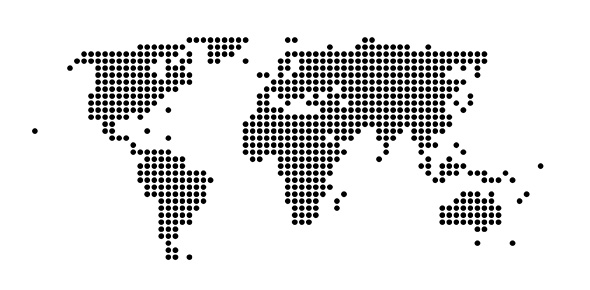# Arch52a - Scale, Scale Factor

14 Questions | Total Attempts: 52SettingsUnderstanding Scale Factor, Model Space and Paper Space relationship, and commands/shortcuts

• 1.
What is model space most used for?
• A.

Drawing vector lines

• B.

Plotting

• 2.
What is Paper Space specifically used for?
• A.

Drawing vector lines

• B.

Plotting

• 3.
What is the shortcut for SCALE command?
• A.

SC

• B.

S

• 4.
What is SCALE FACTOR used for?
• A.

It is used to scale a drawing in Model Space using a scale of choice. i.e. 1/4"=1'-0"

• B.

It is used while in Paper Space to magnify a drawing through a model view window(MVIEW)

• 5.
What are the most commonly used drawing sheet sizes?
• A.

11"x14", 11"x17", 24"x36", 30"x44"

• B.

8-1/2"x11", 11"x17", 22"x34", 30"x42"

• C.

8-1/2"x11",11"x17",30"x44", 24"x36"

• D.

30"x42"(ARCH E), 24"x36"(ARCH D), 18"x24"(ARCH C),11"x17"(ARCH B)

• E.

B and C

• 6.
What is the magic number used with SCALE FACTOR?
• A.

12

• B.

1

• 7.
In Model Space, SCALE FACTOR is a larger number.( i.e. 24, 48, or 96)
• A.

True

• B.

False

• 8.
In Paper Space, SCALE FACTOR is a fractional number followed by the letters xp. (i.e. 1/48xp, 1/24xp)
• A.

True

• B.

False

• 9.
What is the SCALE FACTOR for 1/4"=1'-0" in Model Space?
• A.

1/48xp

• B.

48

• 10.
What is the SCALE FACTOR for 1/8"=1'-0" in Model Space?
• A.

96xp

• B.

96

• 11.
What is the command MV used for?
• A.

It is used to to create a model view window in Model Space.

• B.

It is used to to create a model view window in Paper Space.

• 12.
Can you create a Model View window(MV) in Model Space?
• A.

Yes

• B.

No

• 13.
Which of the following set of Scale Factors is used in the command:Z-C-(pick center point)(type scale factor)-P (to pan image)
• A.

1,2,4,8,12,16,24,48,96,192,384

• B.

1xp,1/2xp,1/4xp,1/8xp,1/12xp,1/16xp,1/24xp,1/48xp,1/96xp,1/192xp

• 14.
Name all the architectural scales used in architectural drawings. The first is 12"=1'-0". Name the rest.
Related TopicsBack to top